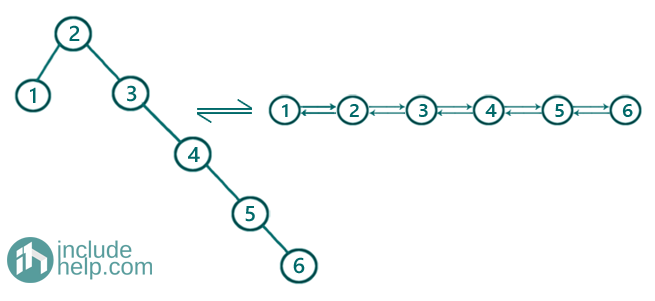# Convert an unbalanced BST to a balanced BST

In this article, we are going to see how to convert an unbalanced BST to a balanced BST?
Submitted by Radib Kar, on October 14, 2020

Earlier, we have talked about what is height-balanced binary search tree & why we need that. In this article, we are going to see how we can convert an unbalanced BST to a balanced BST.

One solution is of course rotation what we do in AVL or other self-balancing trees. The complexity for the rotations is log(n) and in the worst case, we may need n rotations with worst-case complexity O(nlogn) if we solve by rotations. We will see how to convert into a balanced BST with help of rotations while we will discuss AVL and other self-balancing trees.

But there is another way, which requires O(n) extra spaces but worst-case time complexity is O(n) only. Here we discuss this approach only.

For example, the unbalanced BST be the below tree:Obviously, the above tree is a binary search tree but not a balanced one as the left subtree height is only 1 while the right subtree height is 5. So the difference b/w subtree height is 5.

So, to balance is what we do is to just rebuild the BST from scratch. That's why we store the inorder traversal of the unbalanced binary search tree and then start rebuilding a balanced binary search tree.

1) Storing the inorder traversal is pretty straight forward as we need to pass a vector while doing the inorder traversal like below where the vector store is initially empty:

```void inorder(TreeNode* root,vector &store){
if(!root)
return;
inorder(root->left, store);
store.push_back(root->val);
inorder(root->right, store);
}
```

After the traversal stored, it's: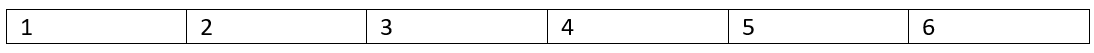2) Constructing height-balanced BST from the inorder traversal

1. Find the middle of the array where we stored the inorder traversal, which is array [first index+ (last index-first index+1)/2]
2. The middle node is the root, left part of the middle node is the left subtree & right part is the right subtree
3. Recursively construct left subtree & right subtree.

Below is the visualization of balancing the BST

Creating the root first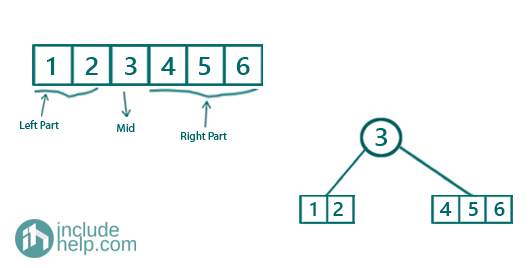Creating the left subtree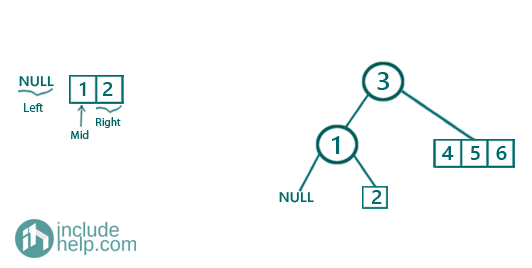Since 2 is the only element in the array it would be the leaf node there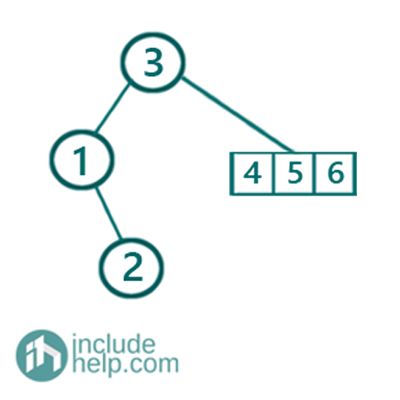Creating the right subtree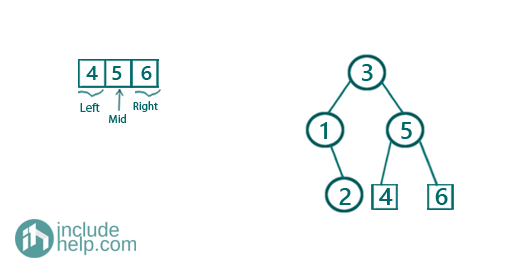So the final tree is: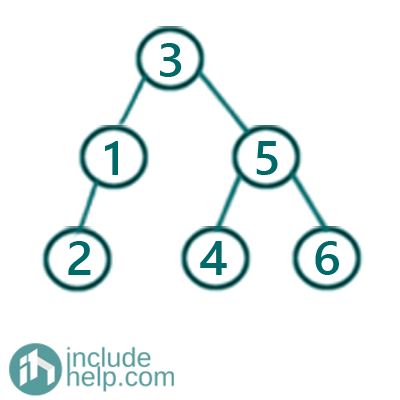Which is a balanced one.

Below is the cpp implementation of the above:

```#include <bits/stdc++.h>
using namespace std;

// tree node is defined
class TreeNode {
public:
int val;
TreeNode* left;
TreeNode* right;
TreeNode(int data)
{
val = data;
left = NULL;
right = NULL;
}
};

//level order traversal for BST
void levelorder(TreeNode* root)
{
if (!root)
return;

queue<TreeNode*> q;
q.push(root);
q.push(NULL);

int count = 1;
cout << "Start of level: " << count << endl;
while (!q.empty()) {
TreeNode* temp = q.front();
q.pop();
if (temp == NULL) {
if (!q.empty()) {
q.push(NULL);
cout << "\nEnd of level: " << count << endl;
count++;
cout << "Start of level: " << count << endl;
}
else {
cout << "\nEnd of level: " << count << endl;
}
}
else {
cout << temp->val << " ";
if (temp->left)
q.push(temp->left);
if (temp->right)
q.push(temp->right);
}
}
}

void inorder(TreeNode* root, vector<int>& store)
{
if (!root)
return;
inorder(root->left, store);
store.push_back(root->val);
inorder(root->right, store);
}

//create a self balanced tree from the sorted array
TreeNode* createBalancedBst(vector<int>& store, int low, int high)
{
if (low > high)
return NULL;
if (low == high)
return new TreeNode(store[low]);

int mid = (low + high) / 2;

TreeNode* root = new TreeNode(store[mid]);
root->left = createBalancedBst(store, low, mid - 1);
root->right = createBalancedBst(store, mid + 1, high);
return root;
}
//helper function to balance the BST
TreeNode* balanceBST(TreeNode* root)
{
vector<int> store;
inorder(root, store);
TreeNode* newroot = createBalancedBst(store, 0, store.size() - 1);
return newroot;
}

int main()
{
//below is the unbalanced BST as per example
TreeNode* root = new TreeNode(2);

root->left = new TreeNode(1);
root->right = new TreeNode(3);
root->right->right = new TreeNode(4);
root->right->right->right = new TreeNode(5);
root->right->right->right->right = new TreeNode(6);

cout << "Level order traversal of the unbalanced BST is :\n";
levelorder(root);

cout << "Balancing the BST ...\n";
TreeNode* newroot = balanceBST(root);

cout << "BST is now balanced...\n";
cout << "Level order traversal of the balanced BST is :\n";
levelorder(newroot);

return 0;
}
```

Output:

```Level order traversal of the unbalanced BST is :
Start of level: 1
2
End of level: 1
Start of level: 2
1 3
End of level: 2
Start of level: 3
4
End of level: 3
Start of level: 4
5
End of level: 4
Start of level: 5
6
End of level: 5
Balancing the BST ...
BST is now balanced...
Level order traversal of the balanced BST is :
Start of level: 1
3
End of level: 1
Start of level: 2
1 5
End of level: 2
Start of level: 3
2 4 6
End of level: 3
```

N.B: Here we have used extra memory to store the unbalanced binary search tree into a vector/array. We can also do it in place without using extra memory. We can convert the Unbalanced BST to a double linked list by just flatting the right & left pointer as next & prev pointer respectively then can construct a height-balanced BST from the doubly linked list. There we have converted a single linked list into a balanced BST, but here you need a bit of modification to work on doubly linked list. The concept would remain the same. Below is the conversion of the BST to a sorted doubly linked list.